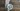# Math.sign：在 Javascript 中如何检查一个数字是正数还是负数

``````const positive = 5;
const negative = -5;
const zero = 0;

Math.sign(positive); // 1
Math.sign(negative); // -1
Math.sign(zero); // 0``````

## Math.sign 返回值

`Math.sign()` 有 5 个可能的返回值。

``````1; // positive number
-1; // negative number
0; // positive zero
-0; // negative zero
NaN; // not a number``````
``````Math.sign(8); // 1
Math.sign(-8); // -1

Math.sign(0); // 0
Math.sign(-0); // -0

Math.sign(NaN); // NaN
Math.sign('hello'); // NaN
Math.sign(); //NaN``````

``````Math.sign(-8);

// ✅ return -1
// ❌ It doesn't return -8``````

## Math.sign 与比较运算符

``````if (number > 0) {
// Positive
} else {
// Negative
}``````

``````if (Math.sign(number) > 0) {
// Positive
} else {
// Negative
}``````

``````const number = 5;

number > 0; // true
Math.sign(number); // 1``````

### 用 Math.sign 解决一个算法挑战

``````Input: 123;
Output: 321;

Input: -123;
Output: -321;``````
``````function reverseInteger(num) {
const numArray = Math.abs(num) // Get the absolute value of our number > 321
.toString() // Convert our number to a string > '321'
.split('') // Convert our string of numbers to an array > [3, 2, 1]
.reverse() // Reverse our array of numbers > [1, 2, 3]
.join(''); // Convert our array back to a string > 123

const sign = Math.sign(num); // -1
return numArray * sign;
// Multiply our reverse string with the sign will produce the correct reverse number
}

reverseInteger(-321); // -123``````

## 负数零

``````Math.sign(0); // 0
Math.sign(-0); // -0``````

## Math.sign 浏览器支持

MDN 上关于 Math.sign 浏览器支持表

### IE 的代码花絮

``````const positive = 5;
const negative = -5;
const zero = 0;

positive === 0 ? positive : positive > 0 ? 1 : -1; // 1
negative === 0 ? negative : negative > 0 ? 1 : -1; // -1
zero === 0 ? zero : zero > 0 ? 1 : -1; // 0``````

### Math.sign 填充

``````if (!Math.sign) {
Math.sign = function (x) {
return (x > 0) - (x < 0) || +x;
};
}``````